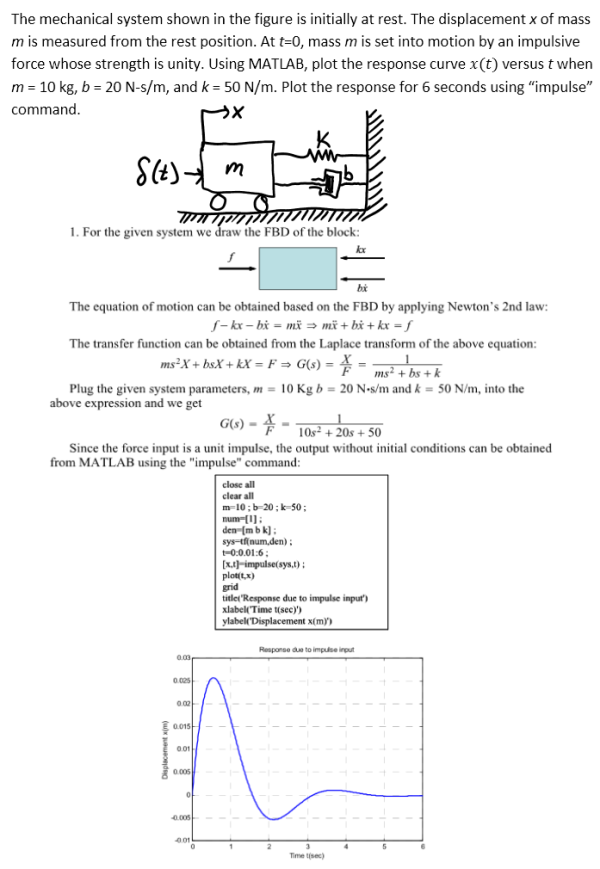The mechanical system shown in the figure is initially at rest. The displacement x of mass m is measured from the rest position. At t=0, mass m is set into motion by an impulsive force whose strength is unity. Using MATLAB, plot the response curve x(t) versus t when m=10 kg, b=20 Ns/m, and k=50N/m. Plot the response for 6 seconds using "impulse" command.The mechanical system shown in the figure is initially at rest. The displacement x of mass m is measured from the rest position. At t=0, mass m is set into motion by an impulsive force whose strength is unity. Using MATLAB, plot the response curve x(t) versus t when m=10 kg, b=20 Ns/m, and k=50N/m. Plot the response for 6 seconds using "impulse" command.

System Dynamics Page 2 dynamics dynamics dynamics dynamics dynamics dynamics dynamics System dynamics Page 3